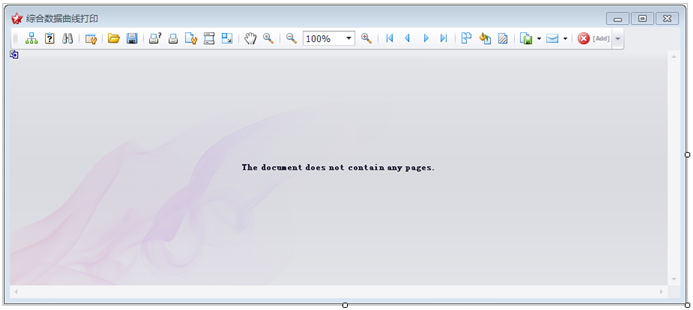# Winform开发框架之数据曲线报表

### 1、按每行一个用户的数据显示曲线报表图形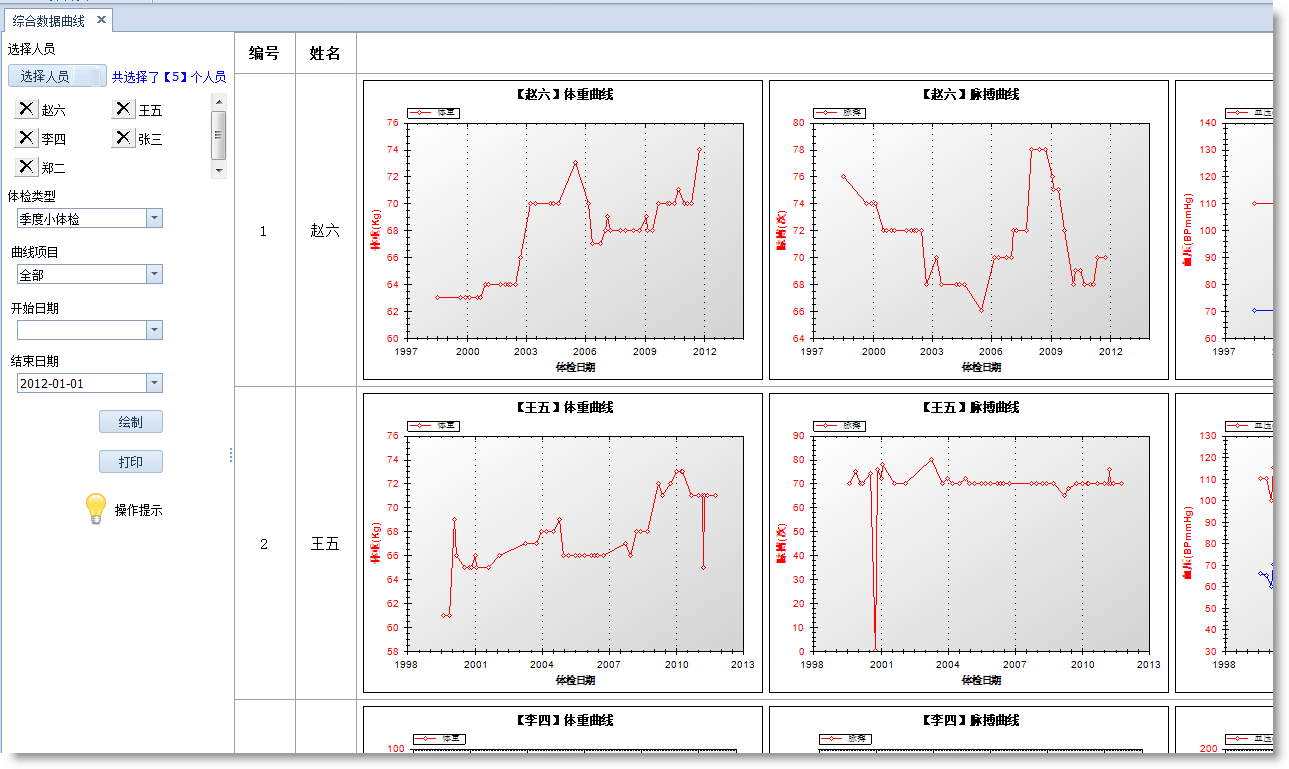### 2、每个曲线图形可以双击打开，进行放大缩小的操作，方便用户查看。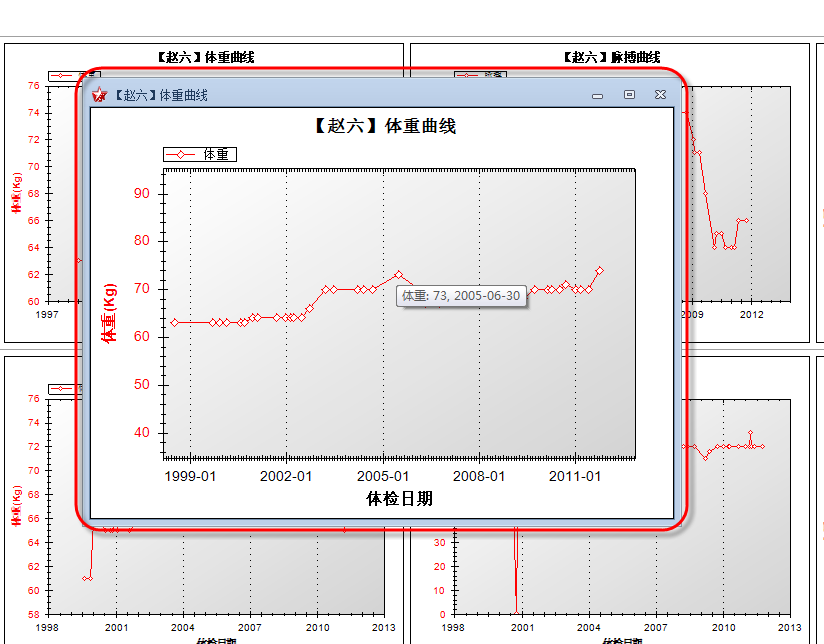### 3、提供自定义打印及文档导出功能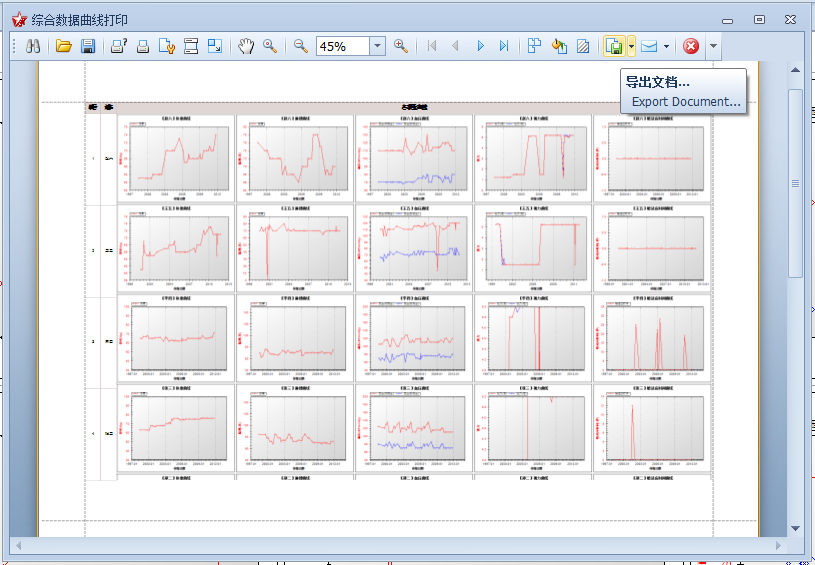### 4、曲线报表具体实现过程及思路

#### 1）设计报表显示主界面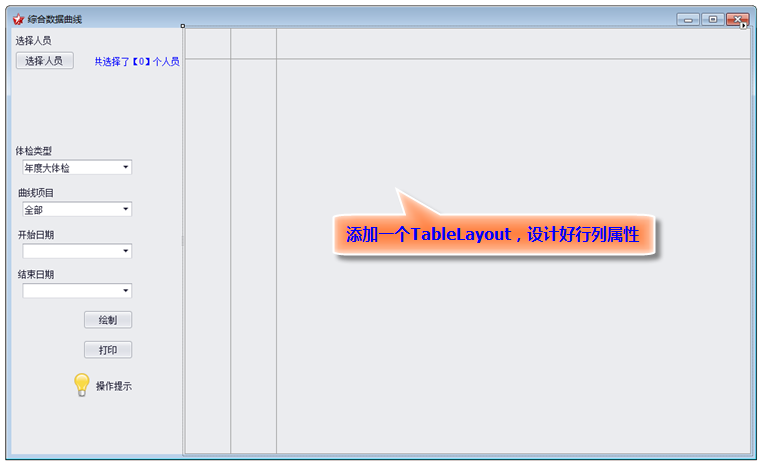#### 2）设计曲线报表组件

public void BindData() { switch (CurveData.CurveType) { case CurveType.体重: BindWeight(); break ; case CurveType.身长: BindHeight(); break ; case CurveType.脉搏: BindPulse(); break ; case CurveType.血压: BindBlood(); break ; case CurveType.视力: BindSight(); break ; case CurveType.暗适应时间: BindDarkAdapTime(); break ; case CurveType.体温: BindTemperature(); break ; case CurveType.全部: #region 全部 if (CurveData.CheckType == CheckType.季度小体检) { BindWeight(); BindPulse(); BindBlood(); BindSight(); BindDarkAdapTime(); } else if (CurveData.CheckType == CheckType.年度大体检) { BindWeight(); BindHeight(); BindPulse(); BindBlood(); BindSight(); } else if (CurveData.CheckType == CheckType.飞行前体检) { BindTemperature(); BindPulse(); BindBlood(); } break ; #endregion } }
private void BindWeight() { DataTable dt = null ; if (CurveData.CheckType == CheckType.年度大体检) { dt = BLLFactory<LargeCheckSurgical> .Instance.GetWeightData(CurveData.StartDate, CurveData.EndDate, CurveData.PilotID); } else if (CurveData.CheckType == CheckType.季度小体检) { dt = BLLFactory<SmallCheck> .Instance.GetWeightData(CurveData.StartDate, CurveData.EndDate, CurveData.PilotID); } WeightCurve curve = new WeightCurve(); curve.CurveData = CurveData; curve.dataTable = dt; this .layoutPanel1.Controls.Add(curve); }

#### 3）设计曲线项目组件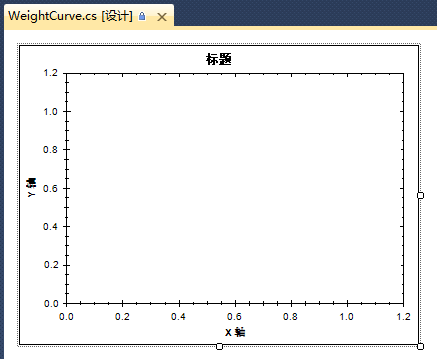GraphPane myPane = zgc.GraphPane; myPane.CurveList.Clear(); // 设置标题及坐标轴的说明 myPane.Title.Text = string.Format( " 【{0}】体重曲线 " , CurveData.PilotName); myPane.XAxis.Title.Text = " 体检日期 " ; myPane.YAxis.Title.Text = " 体重(Kg) " ; PointPairList list = new PointPairList(); foreach(DataRow row in dataTable.Rows) { DateTime checkDate = Convert.ToDateTime(row[ " CheckDate " ].ToString()); double x = ( double) new XDate(checkDate); double y = Convert.ToInt32(row[ " Weight " ].ToString()); list.Add(x, y); } LineItem myCurve = myPane.AddCurve( " 体重 " , list, Color.Red, SymbolType.Diamond); myCurve.Symbol.Fill = new Fill(Color.White);

#### 4）设计图表打印模块

protected virtual void DrawRow(BrickGraphics graph, int rowIndex, int col, Control ctrl, float left) { graph.BackColor = Color.White; RectangleF bounds = new RectangleF(left, 0 , ctrl.Width, ctrl.Height); bounds.Y = (rowIndex - 1) * bounds.Height; if (ctrl is Label) { TextBrick brick = graph.DrawString(ctrl.Text, bounds); brick.HorzAlignment = DevExss.Utils.HorzAlignment.Center; brick.VertAlignment = DevExss.Utils.VertAlignment.Center; const int LeftPadding = 4 ; brick.Padding = new PaddingInfo(LeftPadding, brick.Padding.Right, brick.Padding.Top, brick.Padding.Bottom); } else { int width = ctrl.Size.Width; int height = ctrl.Size.Height; Bitmap bm = new Bitmap(width, height); ctrl.DrawToBitmap(bm, new Rectangle( 0, 0 , width, height)); ImageBrick brick = graph.DrawImage(bm, bounds); brick.SizeMode = ImageSizeMode.ZoomImage; } }